## Example Questions

← Previous 1 3 4 5 6 7

### Example Question #1 : Proportion / Ratio / Rate

A solution made up ofalcohol by volume is mixed withliters of solution that isalcohol by volume. How much, in liters, of thealcoholic solution is needed to make a mixture that isalcohol by volume?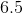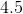Explanation:

Letrepresent the number of liters of the 40% solution. Then it follows thatliters of the 40% solution plus 4 liters of the 10% solution will equal (x+4) liters of a 25% solution. This can be represented by the following equation: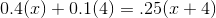Now solve for x: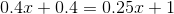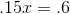You will need 4 liters of the 40% solution in order to make a mixture that is 25% alcohol by volume

### Example Question #2 : Proportion / Ratio / Rate

A solution isparts water,parts wine, andpart honey.  If a container of this solution containsgallons of water, how much total solution is there in it?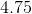gallons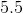gallonsgallonsgallonsgallonsgallons

Explanation:

To begin, notice that there is a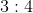ratio between the water in your container and the water specified by the mix of the components.  Given that there aretotal parts in your solution, this means that you can set up this equation: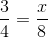Multiplying both sides by 8, you get: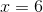There aretotal gallons of solution.

### Example Question #3 : Proportion / Ratio / Rate

A solution is made up ofparts water,parts orange juice, andparts apple juice.  If you wanted the percentage of orange juice to be% of the solution, how many parts would you need to add?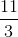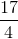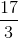Explanation:

To begin, you know that the basic form of the solution has a total of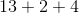, orparts.  Now, we know that we are going to have to addparts of orange juice.  This means that the new solution will have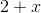parts orange juice and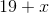total parts (since we are adding to the original).  Since we want this to be% orange juice, we really want the following equation to be true: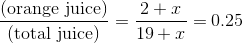Solve the following equation, therefore: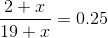Multipy by: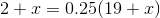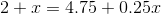Now, isolate: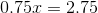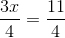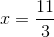### Example Question #1 : Proportion / Ratio / Rate

Flour, eggs, sugar, and chocolate chips are mixed by weight in the ratio of 12 : 5 : 3 : 5, respectively. How many pounds of chocolate chips are there in 75 pounds of the mixture?

5

18

15

25

15

Explanation:

First, add up the four parts of the ratio. This equals 25 parts. These 25 parts make up the 75 pound mixture, which means the 75 pound mixture is composed of 3 times the 25 parts (25x = 75 so x = 3).

This allows you to know that the number of pounds of chocolate chips is 3 times the ratio, i.e. 3 * 5 = 15. The mixture includes 15 pounds of chocolate chips. The answer is 15.

### Example Question #5 : Proportion / Ratio / Rate

If there are 120 men and women on a committee, and the ratio of men to women is 5 : 1, how many more men are on the committee than women?

72

80

96

100

80

Explanation:

The best approach is to add the numerator and denominator of the ratio (5 + 1 = 6), and then divide the total by that sum (120/6 = 20). This gives you the value for 1 part when the total is divided into 6 parts and, luckily for us, there is only 1 part women for every 5 parts men. Now set up equal ratios: men/women = 5/1 = x/20. Solve for the number of men by cross multiplying (5 * 20 = 1x). So there are 100 men and 20 women, which makes 80 more men than women.

### Example Question #2 : Proportion / Ratio / Rate

You have a rope of some length, but 2/3rds of it is cut off and thrown away.  1/4th of the remaining rope is cut off and thrown away. What proportion of the original rope remains?

The answer cannot be determined from the given information.

1/10

1/3

1/4

1/6

1/4

Explanation:

If 2/3 is cut off and thrown away, that means 1/3 of the original length remains. Of this, 1/4 gets cut off and thrown away, meaning 3/4 of 1/3 still remains. Multiplying 3/4 * 1/3, we get 1/4.

### Example Question #7 : Proportion / Ratio / Rate

If the sum of a, b and c is 400, and a is 1/3 b and b is  1/4 c, what is the value of a?

66

33

20

300

25

25

Explanation:

Explanation: For this type of problem, build an equation that represents the relationships between the quantities and solve for the quantitiy you need. The problem states that b=1/4c and a = 1/3b. Thus, a = 1/3(1/4)c, or 1/12 of c. Now put everything in terms of c, thus 1/12c + 1/4c +c = 400. Now comes the tricky step--combine like terms and create the improper fraction (1/12c + 3/12c + 12/12c = 16/12c). Reduce the fraction to 4/3. So 400 is 4/3 of c. Thus c is 3/4 of 400, or 300. a is 1/12 of 300, or 25.

### Example Question #8 : Proportion / Ratio / Rate

Erin went to the movies with her friends.  She spent 1/4 of her allowance on movie tickets and 3/5 of the remaining money on popcorn.  If her allowance is $10, how much money remains? Possible Answers:$1.50

$3.00$8.50

$7.00$5.00

$3.00 Explanation: Since she spent 1/4 on the ticket, 3/5 of the remaining 3/4 of the money was spent on popcorn: 3/5 x 3/4 = 9/20. This means 9/20 of the money was spent on popcorn so in total: 1/4 + 9/20 = 14/20 = 7/10 of her money was spent. This leaves 3/10 of her money behind: 3/10x 10 = 3.00. ### Example Question #9 : Proportion / Ratio / Rate Fudge sells at$18.50 for 5 pounds.  What is the cost for 2 pounds?

$7.40$5.50

$9.25$6.75

$3.70 Correct answer:$7.40

Explanation:

Set up a proportion: 18.50/5 = x/2.  Cross multiply and solve for x: 37 = 5x.... x = 7.40.

### Example Question #10 : Proportion / Ratio / Rate

3 men can paint 3 rooms in 3 hours. How long would it take 1 man to paint 1 room?

1.5 hours

1 hour

3 hours

2 hours

2.5 hours

3 hours

Explanation:

It's tempting to pick 1 hour, but that is a trick answer! Picture 3 men each painting 1 room. All 3 of the rooms are done after 3 hours, so each man actually spends 3 hours painting his room, not 1 hour.

← Previous 1 3 4 5 6 7

Tired of practice problems?

Try live online GRE prep today.